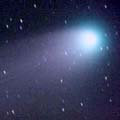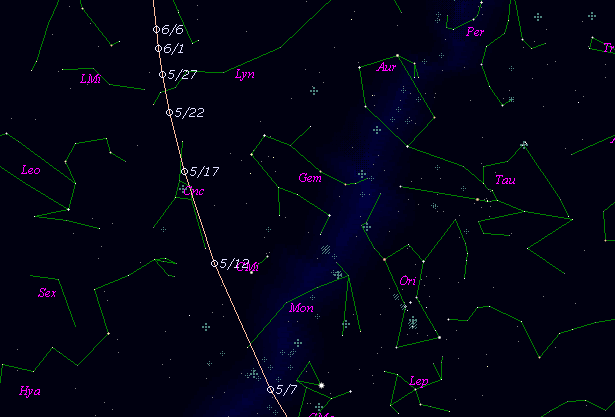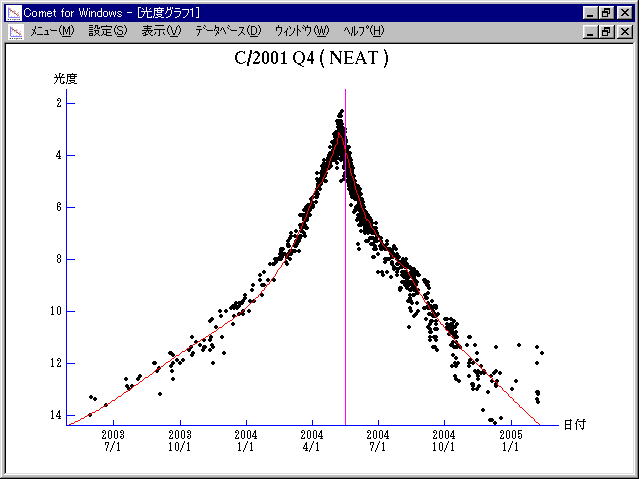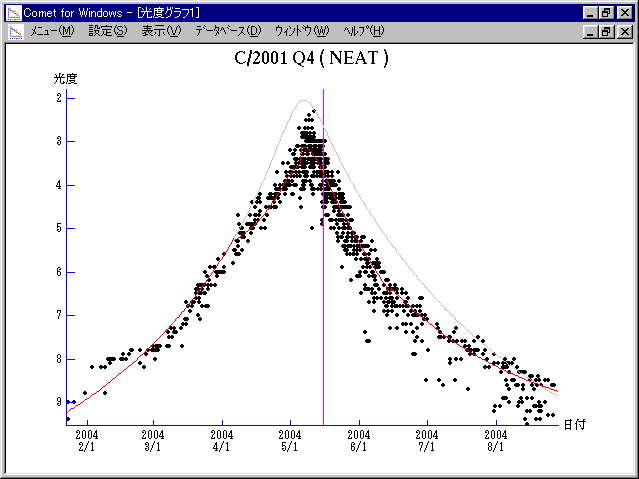# C/2001 Q4 ( NEAT )###Profile

 Discovery Date August 24, 2001 Magnitude 20.0 mag Discoverer Near-Earth-Asteroid Tracking Team (Jet Propulsion Laboratory)

###Pictures###Orbital Elements

```Epoch 2004 June 4.0 TT = JDT 2453160.5
T 2004 May 15.96533 TT                                  MPC
q   0.9619569            (2000.0)            P               Q
z  -0.0006904      Peri.    1.20383     -0.86516743     -0.06629308
+/-0.0000040      Node   210.27863     -0.46794807     -0.24966639
e   1.0006642      Incl.   99.64255     -0.18030507     +0.96606000
>From 1106 observations 2001 Aug. 24-2004 June 11, mean residual 0".77.  Non-
gravitational parameters A1 = +1.49 +/- 0.03, A2 = -0.2965 +/- 0.0282.
```

###Finding Charts###Magnitudes Graph

```        m1 = 3.5 + 5 log d + 10.0 log r  [    ,-400]  (             - 2003 Apr. 11)
m1 = 4.6 + 5 log d +  8.6 log r  [-400, -40]  (2003 Apr. 11 - 2004 Apr.  5)
m1 = 5.9 + 5 log d -  8.5 log r  [ -40, -10]  (2004 Apr.  5 - 2004 May   5)
m1 = 5.7 + 5 log d + 10.0 log r  [ -10,  30]  (2004 May   5 - 2004 June 14)
m1 = 5.8 + 5 log d +  4.0 log r  [  30,  85]  (2004 June 14 - 2004 Aug.  8)
m1 = 3.5 + 5 log d + 14.0 log r  [  85, 160]  (2004 Aug.  8 - 2004 Oct. 22)
m1 = 4.6 + 5 log d + 11.5 log r  [ 160, 400]  (2004 Oct. 22 - 2005 June 19)
m1 = 9.2 + 5 log d +  5.0 log r  [ 400,    ]  (2005 June 19 -             )

* Gray curve is:  m1 = 4.6 + 5 log d + 8.6 log r
```##### The orbital elements are published on M.P.E.C. 2004-L37. The charts are made with StellaNavigator Ver.2.0 for Windows (AstroArts / ASCII). The magnitudes graphs are made with Comet for Windows.